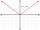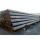# Hyperbola equation

Find the hyperbola equation with the center of S [0; 0], passing through the points:
A [5; 3] B [8; -10]

Result

f = (Correct answer is: f = 7 * x^2 - 3y^2 = 148)#### Solution:Leave us a comment of example and its solution (i.e. if it is still somewhat unclear...):

Showing 0 comments:Be the first to comment!#### To solve this example are needed these knowledge from mathematics:

Looking for help with calculating roots of a quadratic equation? Do you have a linear equation or system of equations and looking for its solution? Or do you have quadratic equation?

## Next similar examples:

1. Expression with powersIf x-1/x=5, find the value of x4+1/x4
2. Quadratic equationFind the roots of the quadratic equation: 3x2-4x + (-4) = 0.
3. Evaluation of expressionsIf a2-3a+1=0, find (i)a2+1/a2 (ii) a3+1/a3
4. Geometric seqFind the third member of geometric progression if a1 + a2 = 36 and a1 + a3 = 90. Calculate its quotient.
5. AlgebraX+y=5, find xy (find the product of x and y if x+y = 5)
6. Null pointsCalculate the roots of the equation: ?
7. Ball gameRichard, Denis and Denise together scored 932 goals. Denis scored 4 goals over Denise but Denis scored 24 goals less than Richard. Determine the number of goals for each player.
8. Square root 2If the square root of 3m2 +22 and -x = 0, and x=7, what is m?
9. EquationEquation ? has one root x1 = 8. Determine the coefficient b and the second root x2.
10. Reciprocal equation 2Solve this equation: x + 5/x - 6 = 4/11
11. RootsDetermine the quadratic equation absolute coefficient q, that the equation has a real double root and the root x calculate: ?
12. Solve 3Solve quadratic equation: (6n+1) (4n-1) = 3n2
13. DiscriminantDetermine the discriminant of the equation: ?
14. Solve equationsolve equation: ?
15. TubesIron tubes in the warehouse are stored in layers so that each tube top layer fit into the gaps of the lower layer. How many layers are needed to deposit 100 tubes if top layer has 9 tubes? How many tubes are in bottom layer of tubes?
16. Linsys2Solve two equations with two unknowns: 400x+120y=147.2 350x+200y=144
17. Theorem proveWe want to prove the sentense: If the natural number n is divisible by six, then n is divisible by three. From what assumption we started?